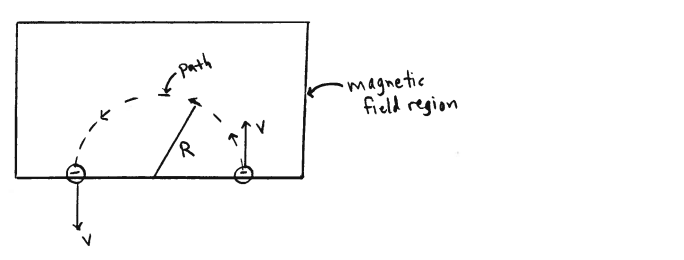Problem: In the rectangular region shown in the sketch there is a uniform magnetic field. A small particle with mass m = 4.0 x 10-12 kg and negative charge q = - 8.0 x 10 -6 C enters the field with velocity v = 5.0 x 104 m/s in the direction shown. The particle travels with constant speed along the semicircular path shown in the sketch. The radius of the path is R = 0.40 m. What are the magnitude and direction of the magnetic field in the region?

FREE Expert Solution
80% (404 ratings)
Problem Details

In the rectangular region shown in the sketch there is a uniform magnetic field. A small particle with mass m = 4.0 x 10-12 kg and negative charge q = - 8.0 x 10 -6 C enters the field with velocity v = 5.0 x 104 m/s in the direction shown. The particle travels with constant speed along the semicircular path shown in the sketch. The radius of the path is R = 0.40 m. What are the magnitude and direction of the magnetic field in the region?